Eigen value is very important for many applications in Science and Engineering such as solution to linear and non-linear differential equations, boundary value problem, chaos theory, discrete dynamical system, Markov chain, network analysis, Population growth model, are just a few applications to mentions. Eigen value of symmetric matrix appears to be very useful for many practical problems. Many statistical and decision-making tools such as multidimensional scaling (MDS) and Principle component Analysis (PCA) are rely heavily on the finding of Eigen value of symmetric matrix of covariance or correlation matrix.

Many people believe that finding Eigen value is very difficult problem. In this tutorial, we will learn an easy way to find Eigen value of a symmetric matrix using MS Excel Goal seeks. In principle, finding Eigen value is the same problem as finding a root of polynomial equation.

Symmetric matrix has special properties that the Eigen values are always real number (not complex number). That is why we can easily solve using MS excel Goal seeks . You can use the procedure below to find the Eigen value of any square matrix, but some of the complex Eigen value may never be found. Click here for explanation on how to get symmetric matrix from any matrix .

Suppose we have a symmetric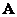, and we want to find the Eigen value of it. The procedure is back to the definition of Eigen value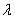as the root of characteristic equation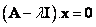, where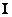is identity matrix and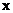is the Eigen vector of matrix. The characteristic equation can be solved if the determinant of the first term is equal to zero, or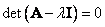. This is exactly what we are going to do in the following steps to find the Eigen value of symmetric matrix . You may download the spreadsheet in here .

1.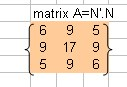Suppose we have the following symmetric matrixas shown in the figure on the right. Optionally, you can give name to the matrix using menu Insert-Name-Define matrix_A , then press OK button
2.Type the identity matrix, which has the same size of matrix. Name it as matrix_I
3. In an empty cell type any number as the first guess of Eigen value, and name it lambda
4.In a new empty cell, type this formula = matrix_A - lambda * matrix_I . Hightlight 3 by 3 cells down and to the right starting from this cell, press F2 and then press CTRL-SHIFT-ENTER together. What you get is matrix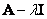. Name this matrix_A_lamdba_I
5. In another cell, type = MDETERM( matrix_A_lamdba_I ) and press Enter
6. From the menu of MS Excel, click Tools-Goal Seek and Goal Seek dialog show up.
7. Set cell contain determinant formula to value 0 ( target value ) by changing cell that contain initial guess of lambda and click OK button. After a few iterations, you get the Eigen value. The correct Eigen value of the matrixin this example is 0.9233, 1 and 27.0767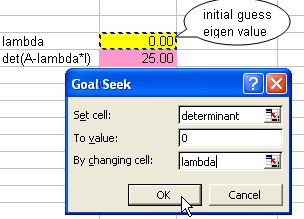Preferable reference for this tutorial is

Teknomo, Kardi (2019). Finding Eigen Value of Symmetric matrix Using Microsoft Excel. https:\\people.revoledu.com\kardi\ tutorial\Excel\EigenValue.html